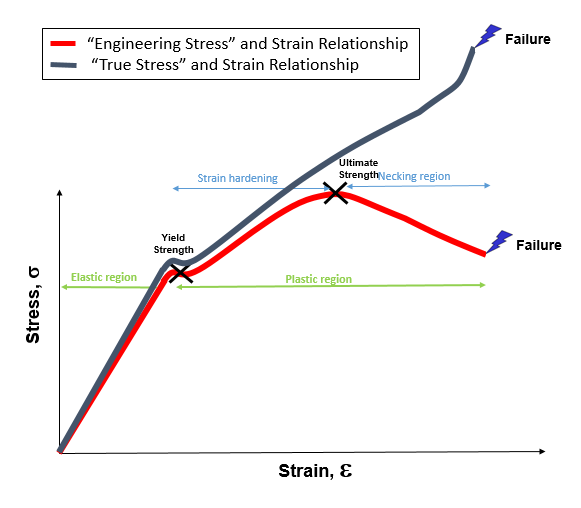Feb 26, 2016

Feb 26, 2016

Feb 26, 2016

# Engineering Stress and Strain Curve DiagramEngineering stress vs strain

Also see True Stress and True Strain

## Engineering Stress

These quantities are defined relative to the original area and length of the specimen. The engineering stress (øe) at any point is defined as the ratio of the instantaneous load or force (F) and the original area (Ao).

• Engineering Stress
• Engineering Strain

## Engineering Strain:

The engineering strain (e) is defined as the ratio of the change in length (L - Lo) and the original length (Lo).
Engineering Strain.

### Engineering Stress And Strain Curve:

Engineering stress-strain curve is obtained from the load-elongation curve. The Yield point, called the yield strength signifies the start of the plastic region.